## Proc logistic rocoptions: problem with contingency table

Hi, I would like to find a threshold with ROC curve.

I have a quantitative variable var1 and a qualitative variable resp_q.

data tab;
input var1 resp_q\$13.;
datalines;
5 Non-répondeur
7 Non-répondeur
7 Répondeur
7 Répondeur
8 Non-répondeur
8 Répondeur
8 Non-répondeur
8 Répondeur
10 Non-répondeur
11 Répondeur
11 Non-répondeur
12 Non-répondeur
13 Répondeur
13 Non-répondeur
13 Répondeur
13 Non-répondeur
14 Répondeur
14 Non-répondeur
16 Non-répondeur
16 Non-répondeur
18 Non-répondeur
;
run;

This is the distribution: proc freq data=tab; table resp_q*var1; run;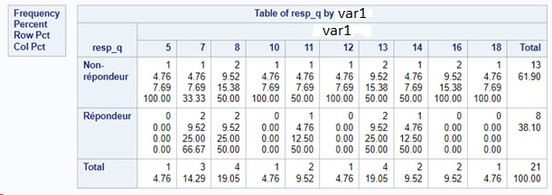Then I do this:

proc logistic data=tab rocoptions(optimal=youden);
model resp_q(event='Répondeur')=var1 / outroc=roc_var1 ;
run;

And this is the output ROC_VAR1: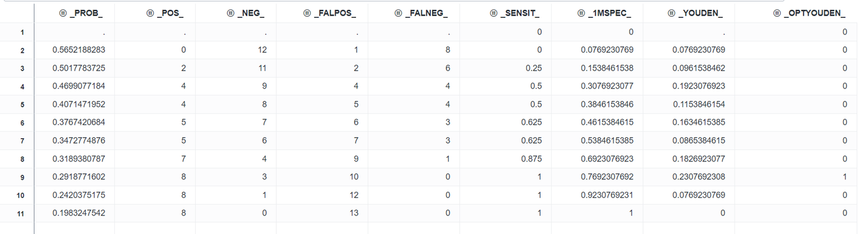I take the row where optyouden=1. This is the 3rd row from the bottom. So, the value corresponding to the threshold is var1=8.

If I calculate my contingency table with the threshold 8, I don't have the same thing that the output.

My contingency table:

 Répondeur Non-répondeur Total T+ 6 11 17 T- 2 2 4 Total 8 13 21

The output ROC_VAR1:

 Répondeur Non-répondeur Total T+ 8 10 18 T- 0 3 3 Total 8 13 21

I don't understand why...

Have you already encountered this problem ?

Thank you.

1 ACCEPTED SOLUTION

Accepted Solutions

## Re: Proc logistic rocoptions: problem with contingency table

The value of your predictor (VAR1) that corresponds to the optimal threshold can be displayed by specifying VAR1 in the ID statement and using ID=ID in ROCOPTIONS:

``````proc logistic data=tab rocoptions(optimal=youden id=id);
id var1;
model resp_q(event='Répondeur')=var1 / outroc=roc_var1 ;
run;
``````

If you do that, you will see in the ROC plot that the optimal threshold corresponds to VAR1=14. You can use that to make a  variable of predicted response levels and produce the 2x2, predicted by actual table:

``````data x; set tab; pred=(var1<=14); run;
proc freq data=x; table pred*resp_q; run;
``````

The resulting table agrees with the _POS_, _NEG_, _FALPOS_, and _FALNEG_ values in the OUTROC= table.

5 REPLIES 5

## Re: Proc logistic rocoptions: problem with contingency table

You do not show us anything related to how you generated

I take the row where optyouden=1. This is the 3rd row from the bottom. So, the value corresponding to the threshold is var1=8.

If I calculate my contingency table with the threshold 8, I don't have the same thing that the output.

My contingency table:

 Répondeur Non-répondeur Total T+ 6 11 17 T- 2 2 4 Total 8 13 21

The output ROC_VAR1:

 Répondeur Non-répondeur Total T+ 8 10 18 T- 0 3 3 Total 8 13 21

So it is pretty hard to say why/why not.

## Re: Proc logistic rocoptions: problem with contingency table

The value of your predictor (VAR1) that corresponds to the optimal threshold can be displayed by specifying VAR1 in the ID statement and using ID=ID in ROCOPTIONS:

``````proc logistic data=tab rocoptions(optimal=youden id=id);
id var1;
model resp_q(event='Répondeur')=var1 / outroc=roc_var1 ;
run;
``````

If you do that, you will see in the ROC plot that the optimal threshold corresponds to VAR1=14. You can use that to make a  variable of predicted response levels and produce the 2x2, predicted by actual table:

``````data x; set tab; pred=(var1<=14); run;
proc freq data=x; table pred*resp_q; run;
``````

The resulting table agrees with the _POS_, _NEG_, _FALPOS_, and _FALNEG_ values in the OUTROC= table.

## Re: Proc logistic rocoptions: problem with contingency table

Thank you for your hopfull help.

I did the same thing with an other example:

``````data tab2;
input var1 resp_q\$13.;
datalines;
3 Non-répondeur
3 Non-répondeur
3 Non-répondeur
3 Répondeur
4 Non-répondeur
4 Non-répondeur
4 Répondeur
5 Non-répondeur
5 Non-répondeur
5 Répondeur
6 Répondeur
6 Répondeur
7 Non-répondeur
7 Non-répondeur
7 Non-répondeur
7 Non-répondeur
7 Non-répondeur
8 Non-répondeur
8 Répondeur
9 Répondeur
11 Répondeur
;
run;

proc logistic data=tab2 rocoptions(optimal=youden id=id);
id var1;
model resp_q(event='Répondeur')=var1 / outroc=roc_var1 ;
run;``````

ROC curve of proc logistic: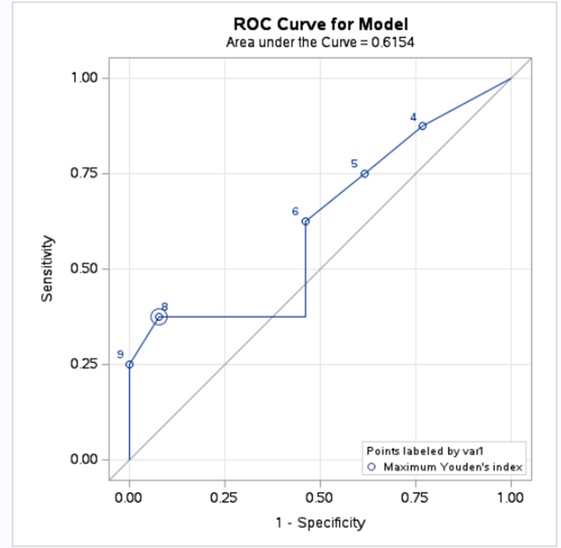The threshold of maximum Youden's index is 8.

Output ROC_VAR1: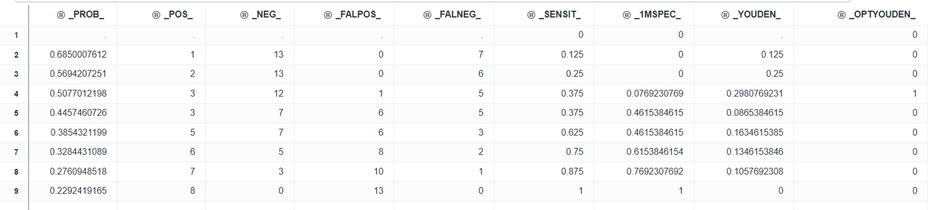If I take the row of maximum Youden's index: _POS_=3, _NEG_=12, _FALPOS_=1 and _FALNEG=5.

Then I do this to verify:

``````data x; set tab2; pred=(var1<=8); run;
proc freq data=x; table pred*resp_q; run;``````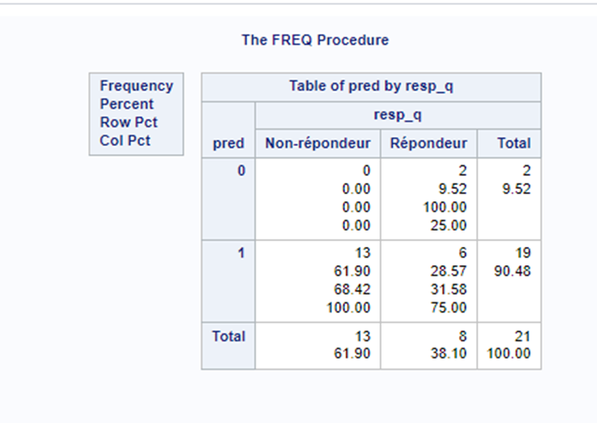The resulting table doesn't agree with the _POS_, _NEG, _FALPOS_, and _FALNEG_ values in the OUTROC=ROC_VAR1.

But if I put ">=8" instead of "<=8", it's good:

``````data x2; set tab2; pred=(var1>=8); run;
proc freq data=x2; table pred*resp_q; run;``````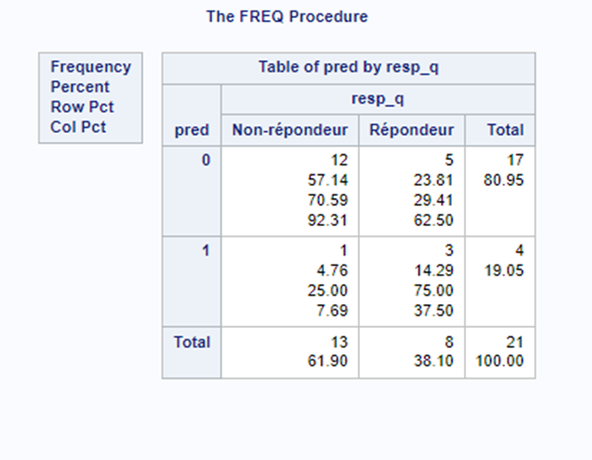Why in the first example I have to use "<=" and in the second ">=" ?

Thank you.

## Re: Proc logistic rocoptions: problem with contingency table

That is because the parameter estimate on VAR1 is positive in this example, negative in the previous one.

## Re: Proc logistic rocoptions: problem with contingency table

Thank you very much !
Discussion stats
• 5 replies
• 320 views
• 3 likes
• 3 in conversation